# Introduction

The BT package implements (Adaptive) Boosting Tree for Poisson distributed response variables, using log-link function. When presented with data, the BT package offers the user the ability to build predictive models and explore the influence of different variables on the response, akin to a data mining or exploration task. The built package is based on the original idea proposed by D. Hainaut, J. Trufin and M. Denuit. For more theoretical details, we refer to the following references:

• M. Denuit, D. Hainaut and J. Trufin (2019). Effective Statistical Learning Methods for Actuaries |: GLMs and Extensions, Springer Actuarial.
• M. Denuit, D. Hainaut and J. Trufin (2020). Effective Statistical Learning Methods for Actuaries ||: Tree-Based Methods and Extensions, Springer Actuarial.
• M. Denuit, D. Hainaut and J. Trufin (2019). Effective Statistical Learning Methods for Actuaries |||: Neural Networks and Extensions, Springer Actuarial.
• M. Denuit, D. Hainaut and J. Trufin (2022). Response versus gradient boosting trees, GLMs and neural networks under Tweedie loss and log-link, Scandinavian Actuarial Journal 2022, 841-866.
• M. Denuit, J. Huyghe and J. Trufin (2022). Boosting cost-complexity pruned trees on Tweedie responses: The ABT machine for insurance ratemaking. Paper submitted for publication.
• M. Denuit, J. Trufin and T. Verdebout (2022). Boosting on the responses with Tweedie loss functions. Paper submitted for publication.

We’ll now show how to use the BT package on classical machine learning problem. In particular, insurance related model will be investigated in the following.

Let’s start by importing the BT package.

library(BT)

# Important modeling remark

As previously mentioned, the BT package is only available for Poisson distributed response variable $$Y$$, in a log-link context.

Using offset in the Poisson framework is often required. In the insurance modelling for example, the offset allows to take into account the time exposure-to-risk. It therefore helps to align the asked premium with respect to the contract duration.

Regarding the BT package, the weighted approach was favored in place of the offset one. In fact, the two are equivalent given some adjustments. More precisely, the modeler is allowed to work either with

• the observed claim count $$Y$$ with offset $$ln(d)$$ under log link, where $$d$$ is the exposure to risk, generally measured in time units, sometimes in distance traveled or other meaningful unit depending on the application, or
• the observed claim frequency (also called claim rate) $$\tilde{Y} = Y/d$$ provided the weight $$\nu = d$$ enters the analysis. In fact, the distribution of the claim frequency $$\tilde{Y}$$ still belongs to the Tweedie family and is called the Poisson rate distribution.

We refer to the first book aforementioned (p. 123) for a detailed proof.

We now focus on the impact of such implementation choice on the Boosting Tree Algorithm. First of all, let us remind the algorithm in our Poisson log-link framework. Given a training set

$\mathcal{D} = \Bigl\{ (d_i, y_i, \mathbf{x}_i), i \in \mathcal{I} \Bigl\},$

where

• $$d_i$$ the exposure-to-risk (e.g. offset term).
• $$y_i$$ is the observed count variable.
• $$\mathbf{x}_i$$ the corresponding features vector.
• $$\mathcal{I}$$ corresponds to the set of all observations,

the following steps are performed:

1. Initialize the score to $\widehat{\text{score}}_0(x) = \mathop{\mathrm{argmin}}_{\beta} \sum_{i \in \mathcal{I}} L\Bigl(y_i, d_i \exp(\beta) \Bigr).$

2. For $$m = 1$$ to $$M$$ do

2.1. Fit a weak learner, regression tree in our context, $$T(\mathbf{x}; \widehat{\mathbf{a}_m})$$ with $\widehat{\mathbf{a}_m} = \mathop{\mathrm{argmin}}_{\mathbf{a}_m} \sum_{i \in \mathcal{I}} L\biggl(y_i, d_i \exp\Bigl(\widehat{\text{score}}_{m-1}(\mathbf{x}_i) + T(\mathbf{x}_i; \mathbf{a}_m)\Bigr)\biggr),$ where

• $$\mathbf{a}_m$$ gathers the splitting variables and their split values as well as the corresponding observed averages in the terminal nodes, i.e. describes the built tree.
• $$L$$ is the loss function, defined as the Poisson deviance in our approach.

2.2. Update $$\widehat{\text{score}}_m(\mathbf{x}) = \widehat{\text{score}}_{m-1}(\mathbf{x}) + T(\mathbf{x}; \widehat{\mathbf{a}_m})$$.

3. Output $$\widehat{\text{score}}(\mathbf{x}) = \widehat{\text{score}}_M(\mathbf{x}).$$

Suppose that we’re at the $$m$$th boosting iteration, the algorithm then fits a weak learner $$T(\mathbf{x}; \widehat{\mathbf{a}_m})$$. Using the above trick, for a given observation $$i$$ one can rewrite the optimization step 2.1 as

$L\biggl(y_i, d_i \exp\Bigl(\widehat{\text{score}}_{m-1}(\mathbf{x}_i) + T(\mathbf{x}_i; \mathbf{a}_m)\Bigr)\biggr) = \nu_i L\biggl(\tilde{y}_i, \exp\Bigl(\widehat{\text{score}}_{m-1}(\mathbf{x}_i) + T(\mathbf{x}_i; \mathbf{a}_m)\Bigr)\biggr),$ where $$\nu_i = d_i$$ and $$\tilde{y_i} = \frac{y_i}{d_i}$$. Using the definition of the Poisson deviance $$L$$, one can easily rewrite the second term as:

$\nu_i L\biggl(\tilde{y}_i, \exp\Bigl(\widehat{\text{score}}_{m-1}(\mathbf{x}_i) + T(\mathbf{x}_i; \mathbf{a}_m)\Bigr)\biggr) = \nu_{mi} L(\tilde{r}_{mi}, \exp(T(\mathbf{x}_i; \mathbf{a}_m))),$ with $\nu_{mi} = \nu_i \exp\Bigl(\widehat{\text{score}}_{m-1}(\mathbf{x}_i) \Bigr)$ and $\tilde{r}_{mi} = \frac{\tilde{y}_i}{\exp \Bigl( \widehat{\text{score}}_{m-1}(\mathbf{x}_i) \Bigr)}.$

The $$m$$th iteration of the boosting procedure therefore reduces to build a single weak learner, on the working training set

$\mathcal{D}^{(m)} = \Bigl\{ (\nu_{mi}, \tilde{r}_{mi}, \mathbf{x}_i), i \in \mathcal{I} \Bigl\},$

using the Poisson deviance loss and the log-link function. Going through iterations, the weights are each time updated together with the responses that are assumed to follow Poisson rate distributions.

# Use-case

The goal of this section is to show how the user can work with BT functions and define an optimal model, according to the selected criteria. We also underline that this use-case is a toy example with some limitations such as running-time constraints. However, the same concepts can easily be extended to real-world problems.

## Import database

Let us import the simulated database BT::BT_Simulated_Data. We refer to the specific database documentation for more details.

db <- BT::BT_Simulated_Data

One can then have a look at this database.

str(db)
## 'data.frame':    50000 obs. of  7 variables:
##  $Y : int 0 0 0 0 0 0 0 0 0 0 ... ##$ Gender      : Factor w/ 2 levels "female","male": 1 2 2 2 2 1 2 1 2 1 ...
##  $Age : int 28 62 37 56 36 24 52 33 47 51 ... ##$ Split       : Factor w/ 2 levels "no","yes": 1 2 1 1 1 2 2 1 1 2 ...
##  $Sport : Factor w/ 2 levels "no","yes": 2 1 1 2 2 2 1 1 1 1 ... ##$ ExpoR       : num  0.102 0.712 0.373 0.621 0.106 ...
##  $Y_normalized: num 0 0 0 0 0 0 0 0 0 0 ... head(db) ## Y Gender Age Split Sport ExpoR Y_normalized ## 1 0 female 28 no yes 0.10197573 0 ## 2 0 male 62 yes no 0.71179081 0 ## 3 0 male 37 no no 0.37298030 0 ## 4 0 male 56 no yes 0.62134765 0 ## 5 0 male 36 no yes 0.10604182 0 ## 6 0 female 24 yes yes 0.05905927 0 One can also perform a quick summary summary(db) ## Y Gender Age Split Sport ## Min. :0.00000 female:24898 Min. :18.00 no :24919 no :24995 ## 1st Qu.:0.00000 male :25102 1st Qu.:29.00 yes:25081 yes:25005 ## Median :0.00000 Median :41.00 ## Mean :0.07296 Mean :41.47 ## 3rd Qu.:0.00000 3rd Qu.:53.00 ## Max. :4.00000 Max. :65.00 ## ExpoR Y_normalized ## Min. :0.0000163 Min. : 0.0000 ## 1st Qu.:0.2494463 1st Qu.: 0.0000 ## Median :0.4999763 Median : 0.0000 ## Mean :0.5002706 Mean : 0.1443 ## 3rd Qu.:0.7519584 3rd Qu.: 0.0000 ## Max. :0.9999931 Max. :27.0002 We leave potential descriptive analysis to the interested reader but we note that the global average claim frequency is sum(db$Y)/sum(db$ExpoR) ##  0.1458411 ## Create working datasets As we’re dealing with machine learning models, a classical approach consists in splitting the dataset into two parts, namely: • A training set which will be heavily used to train the different models and will serve for model selection. • A testing set which will be hold off and used at the end to assess generalization performances. In our example, 80% of the total dataset will be placed in the training set and the remaining part in the testing set. One can note that the claims frequency is approximately similar for all the databases. set.seed(404) trainObs <- sample(seq(1, nrow(db)), 0.8 * nrow(db)) trainSet <- db[trainObs, ] testSet <- db[setdiff(seq(1, nrow(db)), trainObs), ] sum(trainSet$Y)/sum(trainSet$ExpoR) ##  0.1457085 sum(testSet$Y)/sum(testSet$ExpoR) ##  0.1463707 ## Boosting Tree (BT) The basic idea behind this algorithm consists in building weak leaners to explain the remaining error, using all the past iterations. It differs from the Gradient Boosting Methods as we’re here boosting the ratios (as previously explained) rather than the pseudo-residuals, using the defined underlying distribution rather than a gaussian approach. In particular, let us remind that the package does not support offset. However, a problem reformulation can be used as explained before. We want to make profit of all explanatory variables. We then define the following model formula that will be heavily used. formFreq <- Y_normalized ~ Gender + Age + Split + Sport ### BT fit and outputs We propose to begin this section by looking on a simple example resulting from a first run. We can then discuss the different available package’s features. We refer to the package documentation ?BT::BT for more details about the arguments of this function. A first BT can be fitted without cross-validation bt0 <- BT(formula = formFreq, data = trainSet, tweedie.power = 1, ABT = FALSE, n.iter = 50, train.fraction = 0.8, interaction.depth = 3, shrinkage = 0.01, bag.fraction = 0.5, colsample.bytree = NULL, keep.data = TRUE, is.verbose = FALSE, cv.folds = 1, folds.id = NULL, n.cores = 1, weights = ExpoR, seed = 4) One can first have a look at the return object. Almost all the parameters that have been used during the call are stored. bt0$call
## BT(formula = formFreq, data = trainSet, tweedie.power = 1, ABT = FALSE,
##     n.iter = 50, train.fraction = 0.8, interaction.depth = 3,
##     shrinkage = 0.01, bag.fraction = 0.5, colsample.bytree = NULL,
##     keep.data = TRUE, is.verbose = FALSE, cv.folds = 1, folds.id = NULL,
##     n.cores = 1, weights = ExpoR, seed = 4)
bt0$distribution ##  1 bt0$BTParams
## $ABT ##  FALSE ## ##$train.fraction
##  0.8
##
## $shrinkage ##  0.01 ## ##$interaction.depth
##  3
##
## $bag.fraction ##  0.5 ## ##$n.iter
##  50
##
## $colsample.bytree ## NULL ## ##$tree.control
## $tree.control$minsplit
##  2
##
## $tree.control$minbucket
##  1
##
## $tree.control$cp
##  -Inf
##
## $tree.control$maxcompete
##  4
##
## $tree.control$maxsurrogate
##  5
##
## $tree.control$usesurrogate
##  2
##
## $tree.control$surrogatestyle
##  0
##
## $tree.control$maxdepth
##  3
##
## $tree.control$xval
##  0
##
##
## attr(,"class")
##  "BTParams"
bt0$keep.data ##  TRUE bt0$is.verbose
##  FALSE
bt0$seed ##  4 # bt0$w / bt0$response / bt0$var.name

A built-in print function is also available. This method prints some of the already presented values.

print(bt0)
## BT(formula = formFreq, data = trainSet, tweedie.power = 1, ABT = FALSE,
##     n.iter = 50, train.fraction = 0.8, interaction.depth = 3,
##     shrinkage = 0.01, bag.fraction = 0.5, colsample.bytree = NULL,
##     keep.data = TRUE, is.verbose = FALSE, cv.folds = 1, folds.id = NULL,
##     n.cores = 1, weights = ExpoR, seed = 4)
## A boosting tree model with Tweedie parameter : 1  has been fitted.
##  50 iterations were performed.
## OOB generally underestimates the optimal number of iterations although predictive performance is reasonably competitive.
##             Using cv_folds>1 when calling BT usually results in improved predictive performance.
## The best out-of-bag iteration was 50.
## The best validation-set iteration was 50.
## There were 4 predictors of which 4 had non-zero influence.

One can have a specific look at the initialization that has been performed via

str(bt0$BTInit) ## List of 3 ##$ initFit         : num 0.145
##  $training.error : num 0.365 ##$ validation.error: num 0.371
##  - attr(*, "class")= chr "BTInit"

If keep.data=TRUE, the different databases with the last evaluation are returned

str(bt0$BTData) ## List of 2 ##$ training.set  :'data.frame':  32000 obs. of  7 variables:
##   ..$Y_normalized : num [1:32000] 0 1.15 0 0 3.33 ... ## ..$ Gender        : Factor w/ 2 levels "female","male": 1 1 1 2 1 1 1 2 2 1 ...
##   ..$Age : int [1:32000] 53 22 22 21 59 64 49 31 61 28 ... ## ..$ Split         : Factor w/ 2 levels "no","yes": 2 2 1 2 1 2 1 2 1 2 ...
##   ..$Sport : Factor w/ 2 levels "no","yes": 1 2 2 1 1 1 1 2 1 1 ... ## ..$ w             : num [1:32000] 0.977 0.869 0.479 0.374 0.3 ...
##   ..$currTrainScore: num [1:32000] -1.99 -1.86 -1.84 -1.84 -1.98 ... ##$ validation.set:'data.frame':  8000 obs. of  7 variables:
##   ..$Y_normalized: num [1:8000] 0 0 0 0 0 0 0 0 0 0 ... ## ..$ Gender      : Factor w/ 2 levels "female","male": 2 2 2 2 1 1 1 2 1 1 ...
##   ..$Age : int [1:8000] 49 27 45 39 28 65 19 21 43 62 ... ## ..$ Split       : Factor w/ 2 levels "no","yes": 1 2 2 2 1 1 1 2 1 2 ...
##   ..$Sport : Factor w/ 2 levels "no","yes": 1 1 2 1 2 2 2 1 1 2 ... ## ..$ w           : num [1:8000] 0.645 0.175 0.625 0.402 0.572 ...
##   ..$currValScore: num [1:8000] -1.98 -1.94 -1.94 -1.99 -1.93 ... ## - attr(*, "class")= chr "BTData" The fitted values (on the score scale) as well as the computed errors across the iterations are available head(bt0$fitted.values, 5)
##  -1.991089 -1.856422 -1.838851 -1.843473 -1.980151
str(bt0$BTErrors) ## List of 3 ##$ training.error  : num [1:50] 0.369 0.365 0.37 0.368 0.37 ...
##  $validation.error: num [1:50] 0.371 0.371 0.371 0.371 0.371 ... ##$ oob.improvement : num [1:50] 2.11e-05 1.09e-05 1.53e-05 5.85e-06 1.44e-05 ...
##  - attr(*, "class")= chr "BTErrors"

Finally, each weak learner (tree) built in the expansion are stored within the following object. Each element corresponds to a specific rpart object.

length(bt0$BTIndivFits) ##  50 # First tree in the expansion. bt0$BTIndivFits[]
## n= 16000
##
## node), split, n, deviance, yval
##       * denotes terminal node
##
## 1) root 16000 5903.5850 1.0208110
##   2) Age>=24.5 13674 4892.0440 0.9677506
##     4) Gender=female 6730 2296.5900 0.8985216
##       8) Sport=no 3374 1103.4690 0.8238464 *
##       9) Sport=yes 3356 1189.9800 0.9744537 *
##     5) Gender=male 6944 2590.6080 1.0350400 *
##   3) Age< 24.5 2326  993.6583 1.3367930 *
bt0$BTIndivFits[]$frame
##      var     n        wt       dev      yval   complexity ncompete nsurrogate
## 1    Age 16000 1168.6784 5903.5849 1.0208112 0.0030290772        3          0
## 2 Gender 13674 1001.3448 4892.0442 0.9677506 0.0008209918        3          1
## 4  Sport  6730  493.1654 2296.5895 0.8985216 0.0005319459        2          2
## 8 <leaf>  3374  247.8532 1103.4687 0.8238464         -Inf        0          0
## 9 <leaf>  3356  245.3122 1189.9804 0.9744537         -Inf        0          0
## 5 <leaf>  6944  508.1794 2590.6078 1.0350402 0.0003220048        0          0
## 3 <leaf>  2326  167.3337  993.6583 1.3367928 0.0003038660        0          0
##        yval2.1      yval2.2
## 1    1.0208112 1193.0000000
## 2    0.9677506  969.0000000
## 4    0.8985216  443.0000000
## 8    0.8238464  204.0000000
## 9    0.9744537  239.0000000
## 5    1.0350402  526.0000000
## 3    1.3367928  224.0000000

### Optimal iterations number

BT_perf function allows the user to determine the best number of iterations that has to be performed. This one also depends on the type of errors that are available/have been computed during training phase.

Depending on the chosen approach, the following methods can be applied to compute the best number of iterations:

• If user wants to use the validation.error, the argmin(BT$BTErrors$validation.error) will be returned as optimal iteration.
• If user wants to use the oob.improvement, the argmin(-cumsum(BT$BTErrors$oob.improvement)) will be returned as optimal iteration. To be precise, the oob.improvement are not used as such but a smoothed version of it.
• If user wants to use the cv.error, the argmin(BT$BTErrors$cv.error) will be returned as optimal iteration.

We refer to the package documentation ?BT::BT_perf for a thorough presentation of this function arguments.

In our specific context, only the OOB improvements and validation errors are available for the given run (no cross-validation performed).

perfbt0_OOB <- BT_perf(bt0, method = "OOB", oobag.curve = TRUE)
## OOB generally underestimates the optimal number of iterations although predictive performance is reasonably competitive.
##             Using cv_folds>1 when calling BT usually results in improved predictive performance.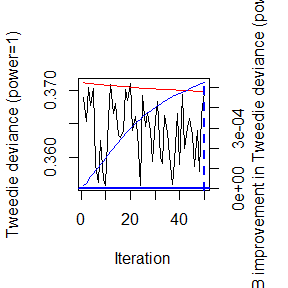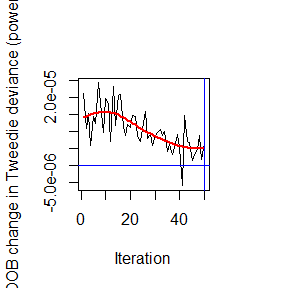perfbt0_OOB
##  50
perfbt0_val <- BT_perf(bt0, method = "validation")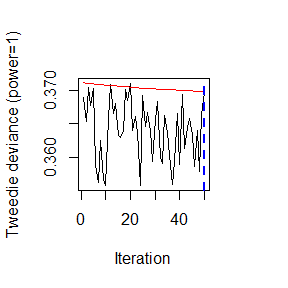perfbt0_val
##  50

Using the implemented “best guess” approach

perfbt0_BG <- BT_perf(bt0, plot.it = FALSE)
## Using validation method...
perfbt0_BG
##  50

### Continue training

It clearly seems that our model does not contain enough weak learners. In fact, the optimal number of iterations is equal to the model number of iterations, meaning that the minimal error (and the related iteration) should still be found. It’s therefore interesting to continue the training.

This training continuation can be performed thanks to the BT_more function. The arguments of this function are explained in ?BT::BT_more and we therefore refer to it for more details.

It will then return a BTFit object (as the BT function does) augmented by the new boosting iterations.

We emphasize that the call to this function can only be made if the original BT call:

• has no cross-validation;
• has been computed with keep.data parameter set to TRUE.
bt1 <- BT_more(bt0, new.n.iter = 150, seed = 4)
# See parameters and different inputs.
bt1$BTParams$n.iter
##  200

It clearly seems that we now reached an optimum.

perfbt1_OOB <- BT_perf(bt1, method = "OOB", plot.it = FALSE)
## OOB generally underestimates the optimal number of iterations although predictive performance is reasonably competitive.
##             Using cv_folds>1 when calling BT usually results in improved predictive performance.
perfbt1_val <- BT_perf(bt1, method = "validation", plot.it = FALSE)
perfbt1_OOB
##  80
perfbt1_val
##  191

### Cross-validation

We often favor doing cross-validation to find the optimal number of iterations. That being said, this approach can be time-consuming and a balance has to be found by the modeler.

Let’s see the results if a 3-folds cross-validation is performed. Please note that the train.fraction is now set to 1 as creating a validation set is less meaningful in the cross-validation context.

bt2 <- BT(formula = formFreq, data = trainSet, tweedie.power = 1, ABT = FALSE, n.iter = 200,
train.fraction = 1, interaction.depth = 3, shrinkage = 0.01, bag.fraction = 0.5,
colsample.bytree = NULL, keep.data = TRUE, is.verbose = FALSE, cv.folds = 3,
folds.id = NULL, n.cores = 1, weights = ExpoR, seed = 4)

Different objects are now available within the new BT results

bt2$cv.folds ##  3 str(bt2$folds)
##  int [1:40000] 3 3 3 3 3 2 1 2 3 2 ...
str(bt2$cv.fitted) ## num [1:40000] -2.06 -1.82 -1.79 -1.68 -2.04 ... str(bt2$BTErrors)
## List of 4
##  $training.error : num [1:200] 0.369 0.371 0.363 0.361 0.355 ... ##$ validation.error: NULL
##  $oob.improvement : num [1:200] 1.88e-05 2.37e-05 2.79e-05 3.01e-05 3.72e-06 ... ##$ cv.error        : num [1:200] 0.366 0.366 0.366 0.366 0.366 ...
##  - attr(*, "class")= chr "BTErrors"

One can also find the optimal number of iterations via

perfbt2_cv <- BT_perf(bt2, method = "cv")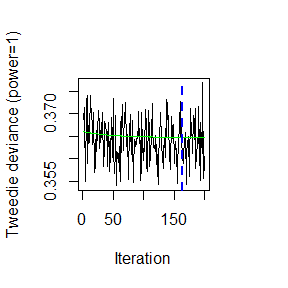### Hyperparameter Optimization

We only worked with one parameter set up to now. In practice, this set has to be found. An usual approach consists in performing a grid search and assessing the performances via cross-validation. Please note that using a validation set can also be used, depending on the computation time.

For this presentation, only one extra boosting tree algorithm will be fitted. In particular, only one different value for interaction.depth will be investigated. In reality the grid search should be way broader, trying multiple multidimensional combinations involving different parameters.

bt3 <- BT(formula = formFreq, data = trainSet, tweedie.power = 1, ABT = FALSE, n.iter = 225,
train.fraction = 1, interaction.depth = 2, shrinkage = 0.01, bag.fraction = 0.5,
colsample.bytree = NULL, keep.data = TRUE, is.verbose = FALSE, cv.folds = 3,
folds.id = NULL, n.cores = 1, weights = ExpoR, seed = 4)

We generally select the best model by finding the one with the lowest cross-validation error.

indexMin <- which.min(c(min(bt2$BTErrors$cv.error), min(bt3$BTErrors$cv.error)))
btOpt <- if (indexMin == 1) bt2 else bt3
perfbtOpt_cv <- BT_perf(btOpt, method = "cv", plot.it = FALSE)

btOpt
## BT(formula = formFreq, data = trainSet, tweedie.power = 1, ABT = FALSE,
##     n.iter = 225, train.fraction = 1, interaction.depth = 2,
##     shrinkage = 0.01, bag.fraction = 0.5, colsample.bytree = NULL,
##     keep.data = TRUE, is.verbose = FALSE, cv.folds = 3, folds.id = NULL,
##     n.cores = 1, weights = ExpoR, seed = 4)
## A boosting tree model with Tweedie parameter : 1  has been fitted.
##  225 iterations were performed.
## OOB generally underestimates the optimal number of iterations although predictive performance is reasonably competitive.
##             Using cv_folds>1 when calling BT usually results in improved predictive performance.
## The best out-of-bag iteration was 113.
## The best cross-validation iteration was 199.
## There were 4 predictors of which 4 had non-zero influence.
perfbtOpt_cv
##  199

### Relative influence

Now that the optimal model has been found, one can compute the relative influence. It corresponds to the gain made by splitting over the features, which is then normalized in order to sum up to 100%.

The summary function allows to compute these values and plot. We refer to this function documentation ?BT:::summary.BTFit for a thorough presentation.

The computation of the relative influence isn’t currently available for the permutation approach. This one should still be developed.

summary(btOpt, n.iter = perfbtOpt_cv)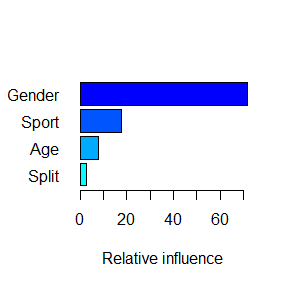##           var   rel_inf
## Age    Gender 71.815815
## Sport   Sport 17.717977
## Gender    Age  7.899891
## Split   Split  2.566317

### Prediction

Fortunately, once a BT object created we can use it to predict on a new database, using the predict function. To this end, the optimal number of iterations is generally a desirable input. We also underline that the model fitted on the whole training set is used to perform these predictions.

The interested reader can find a description of the function arguments in the related documentation ?BT:::predict.BTFit. Please note that if the keep.data argument was set to TRUE and if the newdata is not specified, the prediction will be achieved on the original training set.

We explicit below two usages:

• Prediction (on the link/response scale) using all weak learners up to the best iteration obtained via OOB and CV (it provides 2-dimensional matrix). This is one of the most common option used.
head(predict(btOpt, n.iter = c(BT_perf(btOpt, method = "OOB", plot.it = FALSE), perfbtOpt_cv),
type = "link"), 10)
## As newdata is missing or is not a data frame, the training set has been used thanks to the keep.data = TRUE parameter.
## OOB generally underestimates the optimal number of iterations although predictive performance is reasonably competitive.
##             Using cv_folds>1 when calling BT usually results in improved predictive performance.
##            [,1]      [,2]
##  [1,] -2.036076 -2.073071
##  [2,] -1.795237 -1.778898
##  [3,] -1.791303 -1.765213
##  [4,] -1.723679 -1.685962
##  [5,] -2.030221 -2.062991
##  [6,] -2.030771 -2.066194
##  [7,] -2.030035 -2.058636
##  [8,] -1.900488 -1.881005
##  [9,] -2.006163 -2.020618
## [10,] -1.995247 -2.015617
head(predict(btOpt, n.iter = c(BT_perf(btOpt, method = "OOB", plot.it = FALSE), perfbtOpt_cv),
type = "response"), 10)
## As newdata is missing or is not a data frame, the training set has been used thanks to the keep.data = TRUE parameter.
## OOB generally underestimates the optimal number of iterations although predictive performance is reasonably competitive.
##             Using cv_folds>1 when calling BT usually results in improved predictive performance.
##            [,1]      [,2]
##  [1,] 0.1305400 0.1257988
##  [2,] 0.1660881 0.1688241
##  [3,] 0.1667428 0.1711503
##  [4,] 0.1784085 0.1852661
##  [5,] 0.1313065 0.1270734
##  [6,] 0.1312343 0.1266669
##  [7,] 0.1313309 0.1276280
##  [8,] 0.1494957 0.1524368
##  [9,] 0.1345038 0.1325735
## [10,] 0.1359800 0.1332382
• Prediction (on the response scale) using only the 40th weak learner (tree).
head(predict(btOpt, n.iter = 40, type = "response", single.iter = TRUE), 10)
## As newdata is missing or is not a data frame, the training set has been used thanks to the keep.data = TRUE parameter.
##   0.9192015 0.9192015 0.9192015 1.2280911 0.9192015 0.9192015 0.9192015
##   1.0333582 1.0333582 0.9192015

All the functions available on the classical Boosting Tree side are also available in the Adaptive Boosting Tree context. The only difference lies in the way the number of internal nodes is defined. For a given interaction.depth, ABT will in fact look for the biggest optimal tree having at most interaction.depth internal nodes (i.e. the built weak learner). This idea is basically based on the rpart complexity parameter. Differently said, all the trees in the expansion won’t necessarily contain interaction.depth internal nodes.

By construction, it’s interesting to note that the built trees will converge to a single root node. This can therefore acts as a natural stopping criteria helping to reduce the computation time. However, this option is not implemented in the BT package. Moreover, this behavior is not necessarily observed when random effects (e.g. bag fraction) are used.

### Hyperparameter Optimization

As we did in the BT side, we’ll test two parameters combination and assess their performances via cross-validation. Precisely, values 2 and 3 will be tried out for the interaction.depth parameter. Let’s start by defining the parameters grid thanks to the base::expand.grid function. Once again, we acknowledge that it corresponds to a small representation of real-world problems.

nIterVec <- 225
interactionDepthVec <- c(2, 3)
shrinkageVec <- 0.01
bagFractionVec <- 0.5

gridSearch <- expand.grid(n.iter = nIterVec, interaction.depth = interactionDepthVec,
shrinkage = shrinkageVec, bag.fraction = bagFractionVec)
gridSearch
##   n.iter interaction.depth shrinkage bag.fraction
## 1    225                 2      0.01          0.5
## 2    225                 3      0.01          0.5

We can now loop through all the different scenarios.

abtRes_cv <- list()
for (iGrid in seq(1, nrow(gridSearch))) {
currABT <- BT(formula = formFreq, data = trainSet, tweedie.power = 1, ABT = TRUE,
n.iter = gridSearch[iGrid, "n.iter"], train.fraction = 1, interaction.depth = gridSearch[iGrid,
"interaction.depth"], shrinkage = gridSearch[iGrid, "shrinkage"], bag.fraction = gridSearch[iGrid,
"bag.fraction"], colsample.bytree = NULL, keep.data = FALSE, is.verbose = FALSE,
cv.folds = 3, folds.id = NULL, n.cores = 1, weights = ExpoR, seed = 4)

abtRes_cv[[iGrid]] <- currABT
}

Check that we’ve enough iterations and define the best ABT model.

perfabt1_cv <- BT_perf(abtRes_cv[], method = "cv", plot.it = TRUE)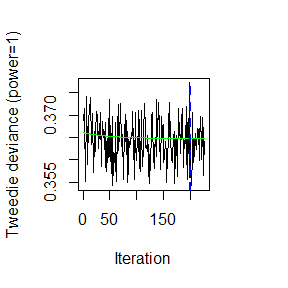perfabt2_cv <- BT_perf(abtRes_cv[], method = "cv", plot.it = TRUE)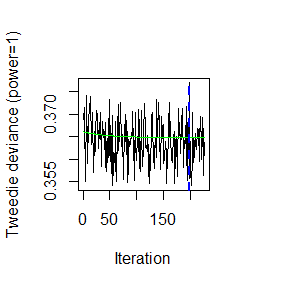We can finally define the best ABT model.

indexMin <- which.min(c(min(abtRes_cv[]$BTErrors$cv.error), min(abtRes_cv[]$BTErrors$cv.error)))
abtOpt <- if (indexMin == 1) abtRes_cv[] else abtRes_cv[]
perfabtOpt_cv <- if (indexMin == 1) perfabt1_cv else perfabt2_cv

abtOpt
## BT(formula = formFreq, data = trainSet, tweedie.power = 1, ABT = TRUE,
##     n.iter = gridSearch[iGrid, "n.iter"], train.fraction = 1,
##     interaction.depth = gridSearch[iGrid, "interaction.depth"],
##     shrinkage = gridSearch[iGrid, "shrinkage"], bag.fraction = gridSearch[iGrid,
##         "bag.fraction"], colsample.bytree = NULL, keep.data = FALSE,
##     is.verbose = FALSE, cv.folds = 3, folds.id = NULL, n.cores = 1,
##     weights = ExpoR, seed = 4)
## An adaptive boosting tree model with Tweedie parameter : 1  has been fitted.
##  225 iterations were performed.
## OOB generally underestimates the optimal number of iterations although predictive performance is reasonably competitive.
##             Using cv_folds>1 when calling BT usually results in improved predictive performance.
## The best out-of-bag iteration was 113.
## The best cross-validation iteration was 199.
## There were 4 predictors of which 4 had non-zero influence.
abtOpt$BTParams$interaction.depth
##  2
perfabtOpt_cv
##  199

## Miscellaneous

Let’s have a look at the resulting weak learners (trees) from BT and ABT expansions.

In the BT case, all the trees contain exactly interaction.depth internal nodes (or splits) whereas in the ABT case one can notice the variation in number of internal nodes (and so the trees’ shapes).

table(sapply(seq(1, perfbtOpt_cv), function(xx) {
nrow(btOpt$BTIndivFits[[xx]]$frame[btOpt$BTIndivFits[[xx]]$frame$var != "<leaf>", ]) })) ## ## 2 ## 199 table(sapply(seq(1, perfabtOpt_cv), function(xx) { nrow(abtOpt$BTIndivFits[[xx]]$frame[abtOpt$BTIndivFits[[xx]]$frame$var != "<leaf>",
])
}))
##
##   0   2
##   4 195

## Models comparison

Once the optimal competing models have been defined, one can assess their generalization performances (i.e. on the test set). To do so, multiple criteria might be used, such as deviance, lift curves, concordance measures, …

Only the first criterion will be investigated for this presentation.

Please note that usually only 1 model is retained beforehand - The test set is not used for model selection. Our specific example remains a case-study!

Let’s start by computing the different model predictions on the test set.

btPredTest <- predict(btOpt, newdata = testSet, n.iter = perfbtOpt_cv, type = "response") *
testSet$ExpoR abtPredTest <- predict(abtOpt, newdata = testSet, n.iter = perfabtOpt_cv, type = "response") * testSet$ExpoR

### Deviance

The deviance is defined as 2 times the log-likelihood ratio of the saturated model compared to the reduced (fitted) one. In other words, it measures the gap between the optimal model and the current one.

devPoisson <- function(obs, pred) {
2 * (sum(dpois(x = obs, lambda = obs, log = TRUE)) - sum(dpois(x = obs, lambda = pred,
log = TRUE)))
}

One can now assess the deviance of the different models.

devPoisson(testSet$Y, btPredTest) ##  3618.013 devPoisson(testSet$Y, abtPredTest)
##  3618.06

For this simulated use-case, it therefore seems that the usual boosting tree approach performs better.